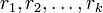# Classification of ambivalent alternating groups

This article classifies the members in a particular group family alternating group that satisfy the group property ambivalent group.

## Statement

The alternating group$A_n$ is an ambivalent group for precisely the following choices of$n$:$n = 1,2,5,6,10,14$.

Note that in the proof, we show that in each of these cases, for every element in the alternating group, there is an element of order two in the alternating group conjugating it to its inverse. Thus, we show that the set of$n$ for which$A_n$ is strongly ambivalent is precisely the same:$n = 1,2,5,6,10,14$.

## Proof

By fact (1), a product of cycles of distinct odd lengths$r_1,r_2,\dots,r_k$ is conjugate to its inverse if and only if$\sum (r_i - 1)/2$ is even. Equivalently, it is conjugate to its inverse if and only if the number of$r_i$s that are congruent to$3$ modulo$4$ is even. Note also that if it is conjugate to its inverse, we can choose as our conjugating element an element of order two: the product of transpositions described above.

### What this boils down to for$n$

Thus, the problem reduces to the following: for what$n$ can we write$n = \sum_{i=1}^k r_i$ in such a way that all$r_i$ are distinct, and the number of$r_i$ that are congruent to$3$ modulo$4$ is odd? These are precisely the$n$ for which$A_n$ is not ambivalent.

We quickly see the following:

•$n = 4d + 3$ can be written in this form, because we can take$k = 1, r_1 = 4d + 3$.
•$n = 4d + 4$ can be written in this form, because we can take$k = 2, r_1 = 4d + 3, r_2 = 1$.
•$n = 4d + 9$ can be written in this form, because we can take$k = 3, r_1 = 1, r_2 = 5, r_3 = 4d + 3$.
•$n = 4d + 18$ can be written in this form, because we can take$k = 4, r_1 = 1, r_2 = 5, r_3 = 9, r_4 = 4d + 3$.

The only cases left are$n = 1,2,5,6,10,14$, and it is readily seen that a decomposition into$r_i$ of the above form is not possible for these$n$.